Mathematical and Physical Journal
for High Schools
Issued by the MATFUND Foundation
 Already signed up? New to KöMaL?# Solutions for advanced problems "A" in October, 2001

In this page only the sketch of the solutions are published; in some cases only the final results. To achieve the maximum score in the competition more detailed solutions needed.

A. 272. The benches of the Great Hall of the Parliament of Neverenough are arranged in a rectangle of 10 rows of 10 seats each. All the 100 MPs have different salaries. Each of them asks all his neighbours (sitting next to, in front of, or behind him, i.e. 4 members at most) how much they earn. They feel a lot of envy towards each other: an MP is content with his salary only if he has at most one neighbour who earns more than himself. What is the maximum possible number of MPs who are satisfied with their salaries?

Solution. We shall show that at most 72 MP's are content with their salaries. Let us represent the members of parliament with a square grid of 10x10 points, and label each point with the salary of the corresponding MP. Let us draw arrows between neighbouring points such that the arrow is directed from the smaller to the larger number: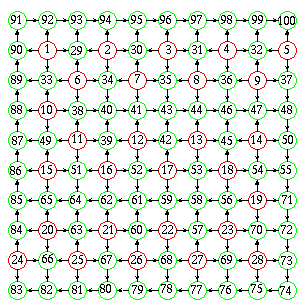(Satisfied MP's are marked in green, and dissatisfied ones in red.)

Let a be the number of satisfied MP's sitting in the corners, b the number of those sitting at the sides of the square, and c the number of those sitting inside.

The number of arrows is 180. There is at most one arrow originating at any satisfied MP, and there will be at least one point where no arrow originates, the MP with the largest salary (obviously satisfied). Hence the number of arrows originating at satisfied MP's is at most a+b+c-1.

There are at most (4-a).2 arrows from the 4-a dissatisfied MP's in the corners, at most (32-b).3 from the 32-b dissatisfied MP's along the sides, and at most (32-b).3 from those (64-c).4 sitting inside. The total number of arrows is thus

180$\displaystyle le$(a+b+c-1)+(4-a).2+(32-b).3+(64-c).4,

that is,

a+2b+3c$\displaystyle le$179.

The one with the lowest salary out of the 36 MP's around the circumference is necessarily dissatisfied, thus a+b35. It is also obvious that a$\displaystyle le$4. By adding the inequalities, we have

3(a+b+c)=(a+2b+3c)+(a+b)+a179+35+4=218,

that is, a+b+c$\displaystyle le$72. Hence, the number of satisfied MP's cannot be greater than 72.

The diagram shows the case when there are exactly 72 MP's who are content with their salaries.

A. 273.  The inscribed circle of triangle ABC touches the sides AB, BC, CA at the points C1, A1, B1 respectively. Given vertex A, line A1B1, and the line joining the midpoint of segment B1C1 to vertex B, construct the triangle ABC with ruler and compasses.

Proposed by: T. Kálmán, Budapest

Solution. Let the angles of the triangle be,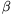and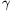, let the centre of the inscribed circle be O, and let F be the midpoint of the segment B1C1. Let M denote the intersection of the lines BF and A1B1, and let N denote the intersection of line A1B1 with the angle bisector AF. First we shall prove that point M lies on the exterior angle bisector from A, or what is equivalent, that the segments AM and B1C1 are parallel.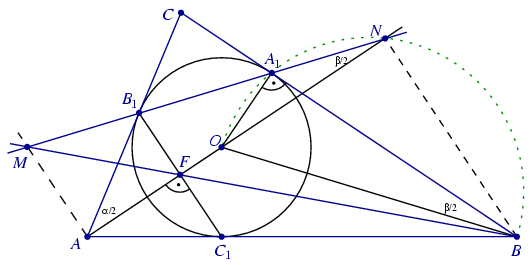By calculating the angles of the triangles A1B1C and AB1N, we have that ANB1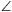=/2. As this is equal to angle OBA1, the quadrilateral OBNA1 is cyclic, and BNO=BA1O=90o. As this is also equal to the angle C1FA, the segments BN and B1C1 are parallel.

It follows from the theorem on parallel transversals, that AF:AN=C1F:BN=FB1:BN=MF:MB, and hence AM és BN are also parallel.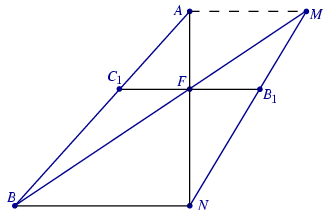Using this information, the steps of the construction are as follows:

1. Construct point M, the intersection of lines AM és BN.

2. Erect a perpendicular at point A to the line AM. This will be the interior angle  bisector that cuts the given lines A1B1 and BF at the points N and F, respectively.

3. Erect a perpendicular at point N to the line AN. This will intersect the line BF at B.

4. B1 is obtained as the intersection of line A1B1 with the perpendicular drawn to AF at point F.

5. Draw a perpendicular to line AB1 at the point B1. This will intersect line AF at O.

6. By reflecting the line AB in the angle bisector BO, obtain the line of side BC. Finally, get the vertex C as the intersection of the line of side BC with the line AB1.

A. 274. Let a, b, c be positive integers for which ac=b2+b+1. Prove that the equation ax2-(2b+1)xy+cy2=1 has an integer solution.

Polish competition problem

Solution 1. The statement of the problem is proved by induction on b. It is allowed that b=0. In that case, a=c=1, and x=y=1 is a solution.

Now suppose that b is positive, and the statement is true for all smaller values of b. The numbers a and c cannot be both greater than b, as when a,cb+1, ac(b+1)2>b2+b+1. As the statement does not change by the interchanging of a and c, we can assume, without restriction, that ab.

Let A=a, B=b-a, C=a-2b+c-1. These are integers, and it is true for them that

B2+B+1=(b-a)2+(b-a)+1=

=(b2+b+1)-2ab+a2-a=

=ac-2ab+a2-a=a(a-2b+c-1)=AC,

and furthermore, A>0, 0$\displaystyle le$B<b, and C=(B2+B+1)/A>0. Hence the induction step can be applied to the numbers A, B, C, which means that there exist the integers X and Y, such that

AX2-(2B+1)XY+CY2=1.

By substituting the definitions of A, B and C, we get

1=AX2-(2B+1)XY+CY2=

=aX2-(2b-2a+1)XY+(a-2b+c-1)Y2=

=a(X+Y)2-(2b+1)(X+Y)Y+cY2.

Therefore, the number pair x=X-Y, y=Y is a solution of the equation.

Solution 2. Consider the set of points in the Cartesian coordinate plane for which ax2-(2b+1)xy+cy2<2. It is easy to show by some calculation that they form an ellipse with an area of $\displaystyle {4\pi\over\sqrt3}$ units.

The elliptical disc is symmetric about the origin, it is convex, and its area is greater than 4 units. Thus, according to Minkowski's theorem there is a mesh point other than the origin in its interior. At that mesh point, the value of ax2-(2b+1)xy+cy2 is between 0 and 2, therefore it is 1.

Remark. In solution 1, we are actually using the transformation (x,y)$\displaystyle mapsto$(x-y,y) to map the elliptical disc onto another ellipse of equal area with smaller coefficients. By repeating the transformation several times, we will always obtain the ellipse x2-xy+y2<2. This ellipse contains six mesh points different from the origin: the points ($\displaystyle pm$1,0), (0,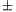1) and (1,1). Therefore, the equation always has six number pairs for solution.